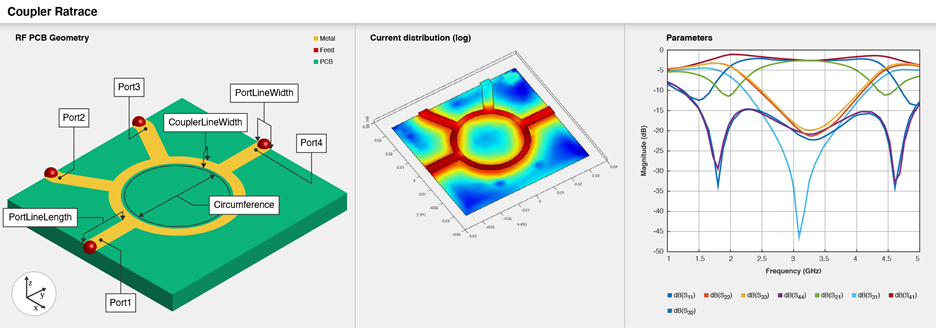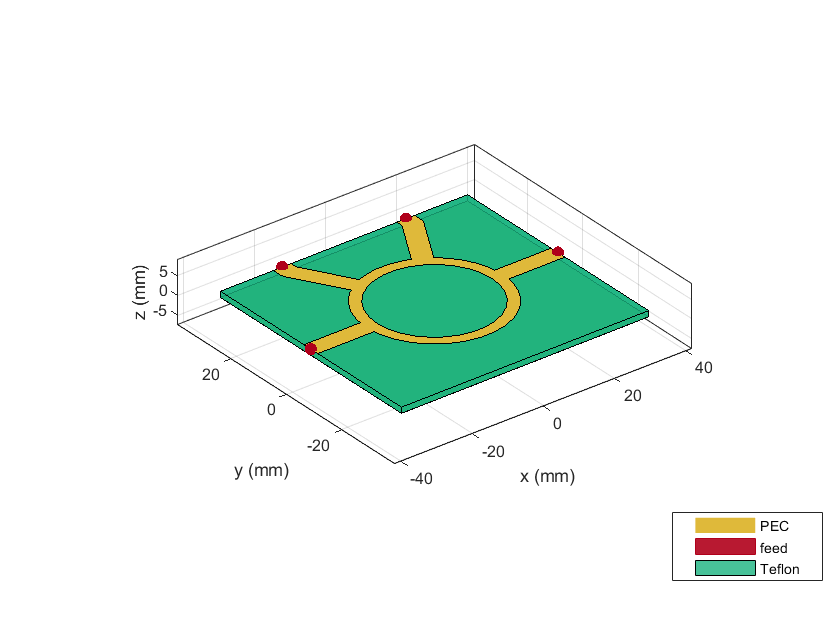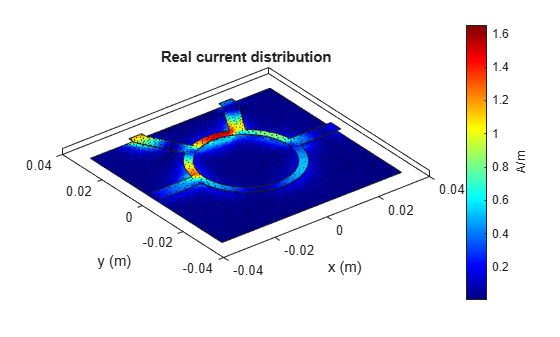# couplerRatrace

Create equal-split rat-race coupler or 180-degree-ring hybrid

Since R2021b

## Description

Use the `couplerRatrace` object to create an equal-split rat-race coupler or a 180-degree-ring hybrid.The rat-race coupler is used as a splitter with a phase shift. When given two inputs, the coupler can create sum and difference ports for added and subtracted power. It also acts as an interface between transmitters and receivers for integrating with an antenna and in building circuits with complex functionality like a comparator with sum and difference ports.

There are four ports and the circumference is 1.5 `λ`. The phase shift between the output ports is 180 degrees. When you apply an input at port 1, port 2 and port 4 are coupled ports, where the output has a phase difference of 180 degrees, and port 3 is the isolated port. When you apply an input at port 3, the output is split equally with same phase at port 2 and port 4.

To analyze the behavioral model for the rat-race coupler, set the `Behavioral` property in the `sparameters` to `true` or 1.

## Creation

### Syntax

``coupler = couplerRatrace``
``coupler = couplerRatrace(Name=Value)``

### Description

example

````coupler = couplerRatrace` creates a rat-race coupler with default property values for a frequency of 3 GHz.```
````coupler = couplerRatrace(Name=Value)` sets Properties using one or more name-value arguments. For example, `couplerRatrace(PortLineLength=0.0286)` creates a rat-race coupler with a port line length of 0.0286 meters. Properties not specified retain their default values.```

## Properties

expand all

Length of the input and the output line in meters, specified as a positive scalar.

Example: `coupler = couplerRatrace(PortLineLength=0.0286)`

Data Types: `double`

Width of the input and the output line in meters, specified as a positive scalar.

Example: `coupler = couplerRatrace(PortLineWidth=0.0070)`

Data Types: `double`

Width of the coupler line in meters, specified as a positive scalar. The default value is for a `λ/4` line with an impedance of `Zo/sqrt(2)` ohms.

Example: `coupler = couplerRatrace(CouplerLineWidth=0.0070)`

Data Types: `double`

Length of the coupler line in meters, specified as a positive scalar. The default value is for a 1.5 `λ` line with an impedance of `Zo/sqrt(2)` ohms.

Example: `coupler = couplerRatrace(Circumference=0.2303)`

Data Types: `double`

Height of the rat-race coupler from the ground plane in meters, specified as a positive scalar.

In the case of a multilayer substrate, you can use the `Height` property to create a rat-race coupler where the two dielectrics interface.

Example: `coupler = couplerRatrace(Height=0.0015)`

Data Types: `double`

Type of dielectric material used as a substrate, specified as a `dielectric` object. The dielectric material in a `couplerRatrace` object with default properties is Teflon. The thickness of the default dielectric material Teflon is `0.0016` m or the same as the `Height` property.

Example: `d = dielectric("FR4");` `coupler = couplerRatrace(Substrate=d)`

Data Types: `string` | `char`

Type of metal used in the conducting layers, specified as a `metal` object. The type of metal in a `couplerRatrace` object with default properties is Copper.

Example: `m = metal("PEC");` `coupler = couplerRatrace(Conductor=m)`

Data Types: `string` | `char`

## Object Functions

 `charge` Calculate and plot charge distribution `coupling` Calculate coupling factor of coupler `current` Calculate and plot current distribution `design` Design rat-race coupler around specified frequency `directivity` Calculate directivity of coupler `feedCurrent` Calculate current at feed port `getZ0` Calculate characteristic impedance of transmission line `isolation` Calculate isolation of coupler `layout` Plot all metal layers and board shape `mesh` Change and view mesh properties of metal or dielectric in PCB component `shapes` Extract all metal layer shapes of PCB component `show` Display PCB component structure or PCB shape `sparameters` Calculate S-parameters for RF PCB objects

## Examples

collapse all

Create and view a default rat-race coupler.

`ratrace = couplerRatrace`
```ratrace = couplerRatrace with properties: PortLineLength: 0.0186 PortLineWidth: 0.0050 CouplerLineWidth: 0.0030 Circumference: 0.1110 Height: 0.0016 Substrate: [1x1 dielectric] Conductor: [1x1 metal] ```
`show(ratrace)`Create a rat-race coupler with default properties.

`coupler = couplerRatrace;`

Set the excitation voltage and the phase angle at the ports of the coupler.

`v = voltagePort(4)`
```v = voltagePort with properties: NumPorts: 4 FeedVoltage: [1 0 0 0] FeedPhase: [0 0 0 0] PortImpedance: 50 ```
`v.FeedVoltage = [1 0 1 0]`
```v = voltagePort with properties: NumPorts: 4 FeedVoltage: [1 0 1 0] FeedPhase: [0 0 0 0] PortImpedance: 50 ```
`v.FeedPhase = [90 0 270 0]`
```v = voltagePort with properties: NumPorts: 4 FeedVoltage: [1 0 1 0] FeedPhase: [90 0 270 0] PortImpedance: 50 ```

Calculate and plot the current on the coupler at 3 GHz.

```figure current(coupler,3e9,Excitation=v,Type='real',Direction='on')```Pozar, David M. Microwave Engineering. 4th ed. Hoboken, NJ: Wiley, 2012.